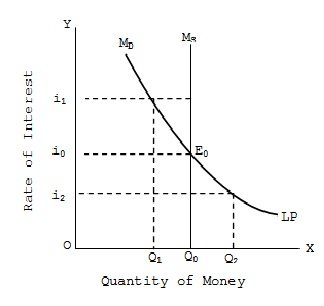Equilibrium Rate of Interest

# Equilibrium Rate of Interest Assignment Help

Home / Macroeconomics Assignment Help / Equilibrium Rate of Interest

# Equilibrium Rate of Interest

Equilibrium rate of interest in determined at that level where demand for money is equal to supply of money.

In fig liquidity preference curve LP shows the money demand (MD)at various interest rates. The supply of money is however fixed at Q0 level as determined by the government policy. The two curves intersect at E0 where demand for money Q0 is equal to supply of money Q0. Thus i0 is the equilibrium interest rate where MD = Ms. If the interest rate is high at i1, then demand for money is Q while supply is Q0 thus giving excess supply Q1 – Q0, since people do not want to hold this excess supply of money with them, they will like to buy bonds, thus, raising bond prices and reducing interest rate back to i0.If the interest rate is low at i2, there is excess demand for money equal to Q0-Q2. Thus, people want to hold more money. They will sell bonds to build up their money balance. The price of bonds to build will fall and the rate of interest goes up to i0. Thus, iO is the only interest rate, at which, demand and supply money are equal and there are no pressure on interest to change in any direction.

### Submit Homework

Submit your homework for a free quote